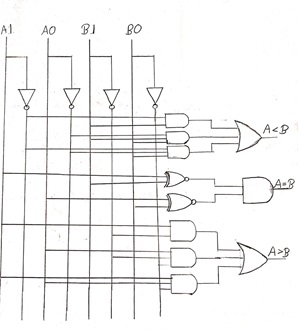# Magnitude Comparator: Types, Applications, and Design

In this tutorial, we will learn about the magnitude comparators in Digital Electronics, the different types of comparators, the designing of 1-bit magnitude comparators, the designing of 2-bit magnitude comparators, and various applications of magnitude comparators. By Saurabh Gupta Last updated : May 11, 2023

## Magnitude Comparator

The word comparator itself means "to compare".

So, what does it compare to? In Digital Electronics, comparators are used to compare two digital and binary numbers to find out which number is greater, smaller, or equal to the other number. Basically, we design a logical circuit that has two inputs (A and B) and three outputs in order to give a result whether a number is equal to another number (A=B) or less than another number (A<B) or greater than another number (A>B).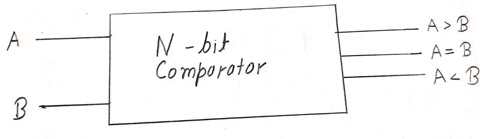## Types of Magnitude Comparator

There are basically four types of magnitude comparators which we study in digital electronics which are listed below:

1. 1-bit magnitude comparator
2. 2-bit magnitude comparator
3. 4-bit magnitude comparator
4. 8-bit magnitude comparator

In this article, we will be reading about the designing process of 1-bit and 2-bit magnitude comparators and later two can be designed following the same principles.

## Applications of Magnitude Comparators

Magnitude comparators are used at several places some of which are listed below:

1. They are used in the Central Processing Unit (CPUs) and Microcontrollers (MCUs).
2. They are used for authorization purposes (such as password management) and biometric applications.
3. They are used to implement controllers and also in servo motors.
4. They are used to address the decoding security of the computers.
5. They are also used in data comparison of variables like temperature, pressure, etc.

## Magnitude Comparators Designing

### 1. Designing of 1-bit Magnitude Comparator

The comparator which is used to compare 2-bits is called a 1-bit magnitude comparator. In this comparator, two inputs are present for a 2-bit numbers and three outputs are present to check whether a number is equal to, less than or greater than the other number.

Now, let us take a look at the truth table of a 1-bit comparator.

From the above truth table, we can see logical expression for each output

expressed as below:

A > B: AB'
A < B: A'B
A = B: A'B' + AB

The expression for A=B is similar to the equation of an EX-NOR Gate. Therefore, A= B can also be represented as A ⨀ B.

Circuit diagram of 1-bit comparator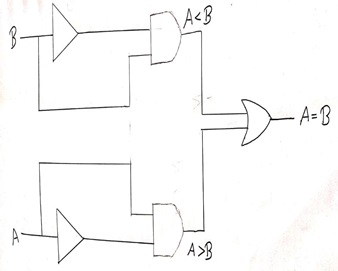### 2. Designing of 2-bit Magnitude Comparator

The comparator which is used to compare to binary number each of 2-bits is called 2-bit magnitude comparator. In these comparators, four inputs and three output are present to check whether a number is equal to, less than or greater than the other number

The truth table for 2-bit comparator can be designed as: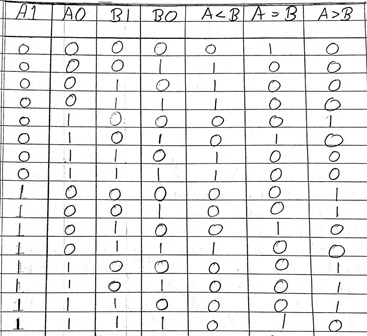By seeing the above truth table K-mapping for following outputs are: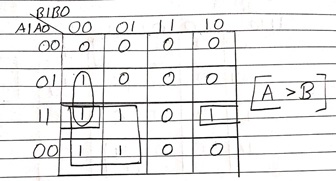Logical expression as derived from the K-Map for A>B can be given as:

A>B = A1B1' + A0B1'B0' + A1A0B0'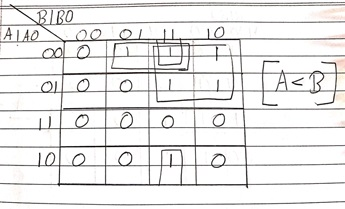Logical expression as derived from the K-Map for A<B can be given as:

A<B = A1'B1 + A0'B1B0 + A1'A0'B0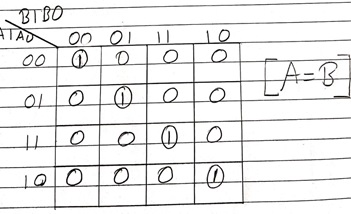Logical expression as derived from the K-Map for A>B can be given as:

A=B: A1'A0'B1'B0' + A1'A0B1'B0 + A1A0B1B0 + A1A0'B1B0'
: A1'B1' (A0'B0' + A0B0) + A1B1 (A0B0 + A0'B0')
: (A0B0 + A0'B0') (A1B1 + A1'B1')
A=B: (A0 ⨀ B0) (A1 ⨀ B1)

Circuit diagram of 2bit comparator